V.5 No 2 1 Supplement 1Supplement 1 On reality of space-time reduction in SR S. B. Karavashkin and O.N. Karavashkina Special Laboratory for Fundamental Elaboration SELF 187 apt., 38 bldg., Prospect Gagarina, Kharkov, 61140, Ukraine phone +38 (0572) 7370624; e-mail: selftrans@yandex.ru , selflab@mail.ru   Discussing our paper “On basic formalism of SR”, adherents of relativistic conception advanced not new but, in their view, important arguments that retain the formalism of SR true, despite the basic discrepancies that we showed in our work. The basic argument of relativists was the events simultaneity and the techniques to indicate it from the view of observers in mutually moving inertial reference frames (IRFs). Mark Alesker formulated the problem of train moving in relation to the platform, as follows. “Let from the centre of wagon moving with the train on rails, there were sent the signals with the limit speed towards the start and finish of the wagon, and these signals switch the lamps there. Then for the passenger these lamps are switched simultaneously, as the distances to them are equal and the speed of signals is same. But for a man on platform this is not so. Because one signal has to catch up with the lamp located in the ‘head’ of wagon, and another signal moves towards the lamp being at rest in the end of wagon. So from the point of a man on platform, the ways of ahead and back signal are different; hence, the time of motion to the lamps is different (with same and limiting speed of signals). So the lamps, as he sees them, will switch at different time”. We agree, this issue is the basic for relativists, the more that in multiple discussions we saw, they do not admit any other techniques to measure the space and time intervals, except of the method on whose basis Einstein developed his theory. And this is regular, as all other techniques, in that number the technique of non-distorted transmission of spatial [1, p. 20] and temporal [2, p. 4] measures shown in our papers, fully destroy the basis of measurements in the SR formalism. However, the methods suggested by Einstein also destroy the basis of SR, and we will show it here. Let us first put in order the relativistic idea of clock synchronisation. Let, in full agreement with the relativistic conception, we have two mutually moving IRFs S and S '. In accordance with this conception, for the clock being at rest in relation to its RF, the synchronisation consists of two stages: The synchronisation of temporal intervals. The synchronisation of absolute numerical values of clock readings. The first stage of synchronisation is determined by the following conditions: “According to the definition, the clocks in A and B will go synchronously, if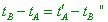(1)
 [3, p. 9], where  tA   is the moment of time in ‘A-scale’ at which the ray is emitted from A to B, tB  is the moment of time in ‘B-scale’ at which the ray is reflected from B, and t'A   is the moment of time in ‘A-scale’ at which the ray is registered at A. “We can give this definition of synchronism in a non-contradictive way and for however much points; thus, the following statements are true: 1. If the clock in B is synchronous with that in A, then the clock in A is synchronous with that in B; 2. If the clock in A is synchronous with that in B and in C, the clocks in B and C go synchronously in relation to each other” [3, p. 9- 10]. With all Einstein’s confidence that his synchronisation technique is complete, further we will see it far from being obvious, but now let us confine ourselves to the statement of the fact and follow the relativistic formulation rigorously. And if we, additionally to this definition, premise the speed of light to be constant and isotropic in all IRFs, the condition (1) will express the relation between the postulate and relativistic definition of time intervals synchronisation. At the second stage of clock synchronisation, relativists establish the interrelation of readings in clocks being stationary with respect to each other and distanced form each other in space. “Now let us premise that the clocks can be checked so that the propagation speed of each ray of light in vacuum measured with these clocks is everywhere equal to the universal constant c under condition that the coordinate system is non-accelerated. Let at the distance r from each other two points A and B provided by the clocks are located at rest in relation to the coordinate system, and let tA  be the reading of clock at A, when to A arrives the light ray propagating through the vacuum in direction AB, and tB be the reading of clock at B at the moment of arriving of light ray to B; then, however the light source, emitted ray and other bodies moved, the equality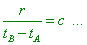(2)
 has always to be true. The amount of readings of all clocks checked so, which we can think to be at rest in relation to the coordinate system and located at the given points of space, we will call the time attributed to the used coordinate system, or, in short, the time of this system” [4, p. 68]. “Keeping this rule, we will yield the time definition in the view of measuring physicist. The event time is just equal to the reading of clock having been put in accordance with the just accepted rule and being at the event point” [5, p. 181]. Again, we yet will not analyse the rigour of relativistic formulation of clock readings synchronisation. We will only mark two points. Firstly, the second stage of clocks synchronisation also is strongly interrelated with the postulate of light speed constancy, and Einstein says of it unambiguously. Secondly, it is important in view of further analysis that the light source, in relativistic idea, can move with respect to the clock at rest, which does not violate the synchronisation. Thirdly, “Generally speaking, the clocks synchronisation is possible also with other techniques: by transferring the clock from one place to another, with help of an elastic constraint etc. We have to require so, lest with such synchronisation to yield any unsolvable discrepancies with the clocks synchronisation through the light signals” [6, p. 22]. This last means that, however we synchronised the clocks, all clocks that are at rest with respect to each other have to be synchronised also in time intervals. And they can be synchronised in readings in several ways, so that they all at their places will independently of each other register the same time, which just will be the time of RF. Thus, “we will call this reference frame, together with the unit scale and clocks that serve to find the time of system, ‘the reference frame S ' ” [4, p. 69]. Now let us move closer to the Mark Alesker’s train. Suppose, in the frame S' moving with the speed v in relation to the stationary RF S, there is located some measurement rod at whose ends and in its middle there are embedded clocks mutually synchronised in S'  . Furthermore, at the middle point C, there is located the source of light pulses that at some moment t'0  emits these signals simultaneously towards the rod ends, as shown in Fig. 1, and these pulses are the short sections of monochromatic EM wave.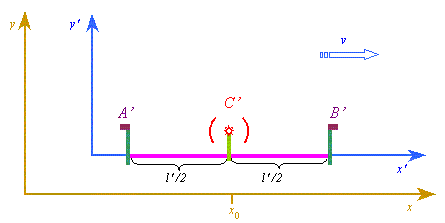Fig. 1. The model of moving rod with the light source in the middle that emits the signals simultaneously to both ends of the rod
 We will be interesting in this problem, whether the intervals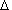tCA  andtCB , as well ast'CA  andt'CB  are equal. To satisfy our interest, let us first lift the standard trick that relativists apply in this case adding to the measurements the time necessary, the ray to achieve straight the observer’s eyes. Our organs of sense are known to be not optimal detectors, they are able to react to far from all kinds of signals. In particular, we are unable to react to the phase of EM wave – of course, if not creating the conditions for an interference. And in this case we will be basing more on the calculation of this pattern made on the basis of wave theory than on the interference pattern as such. The same, it is not necessary for us, the light rays, after they were reflected from A to B, to achieve namely us. We will be satisfied if the wave phase is registered by the synchronised recorders at the selected points of moving RF. We also can be satisfied if at the calculated moments of light arrival to the rod ends, at definite points of stationary RF the observers will be located, which will register the event simultaneity, whether the rod ends will coincide with these points and light signal arrival. This all belongs to the commonly known amount of measurement techniques usually used by experimenters. In other words, if we are aware of the very process, we have not to wait, until the ray informs us of the event but can calculate in full accordance with our knowledge, following all features of the known phenomena, and select the way of registration that provides the least error. In case of Mark Alesker’s train, we can put one observer in the middle of platform to wait the rays arrival. The signal delay will be same. And we can put at definite points of the platform two observers who will register the coincidence. Again, if the phenomena were known, we can recalculate both cases with account of features of the expermental technique. But - recalculated! With it, the experimental results of both techniques will coincide. But if we without calculation, without analysis try operating only with our senses, we will not be able to register the trustworthy event, as it factually will be like taking a desert mirage as a reality. Many travellers who relied not on the knowledge but on the outer perception lost their lives so. ;-) Then we will make use of the fact that, from the relativistic view, the speed of light running from moving source is isotropic in directions and equal to some universal constant c. We will disregard the Doppler effect, as the signal frequency variation is of no significance for the fact of signal arrival to the particular point of space. Finally, we will use one more relativistic premise on whose basis, in particular, the relativistic Doppler effect is calculated. Einstein formulates it so: “Let S  and S ' be the equivalent reference frames, i.e. let these frames have the same-length unit scales and same rate of clocks, meaning that the scales and clocks are compared with each other in the state of relative rest. Then it is obvious that any law of nature in the reference frame S is true in the same form in the frame S ', if S and S ' were at relative rest. The relativity principle requires, this full coincidence of the laws to be extended also to the case when S ' moved directly and uniformly in relation to S. In particular, the light speed in a void in relation to both reference frames has to be expressed by one and the same number” [4, p. 70- 71]. For the source stationary in relation to the moving RF S '  , it is important that in this case “the phase (of the light wave – Authors) has to be invariant” [6, p. 36] for both stationary observer at S and moving observer at S ' at any point of space common for both RFs at a definite moment of time. We will draw our attention to the way to register the equal phases that are the event for both stationary and moving observer. Then, let the source at the point C emits its pulses at the moment when C coincides with the coordinate x0  of the stationary RF. After this, the ray propagates with the constant speed c with respect to the stationary observer. For the ray directed towards the point A (see Fig. 1), the point A, in the view of stationary observer, approaches to the front of ray, so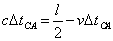(3)
 or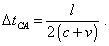(4)
 We would especially mark, we do not refer the ray to the aether and do not introduce that the lengths in moving and stationary RFs have to be certainly equal. The lengths in RFs can be inequal, but ?? = ??  , if A’C’ = B’C’, which follows immediately from Einstein’s transformations. And this is enough, (4) to be true. The same in the view of moving observer, the point B of the rod will ‘run away’ from the wavefront, which means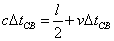(5)
 or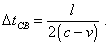(6)
 It is clear that (4) and (6) give the basis for any calculations in the frame of stationary observer, but the main is that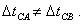(7)
 With it, as we said above, we have no need to add to (4) and (6) the time during which the ray after reaching the rod ends reaches the stationary observer. The stationary observers can be not waiting the ray arrival but observe the coincidence of events - arriving fronts of rays and related rod ends to the certain points of stationary RF. In this case they will register the inequal phases. Hence, the moving observers at the rod ends also have to register the non-simultaneity of wavefront arrival to the rod ends, irrespectively of, whether the rod reduces due to its motion or not, as the coincidence of the rod end and ray front is the event. This result is shown in the animated Fig. 2.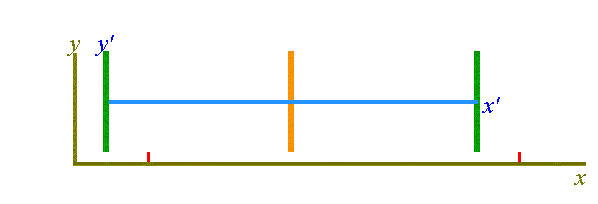Fig. 2. The animation shows the non-simultaneity of arriving wavefront of light to the rod ends; v = 0,2 c
 The animation visually shows that if from the view of stationary observer the speed of light propagation is same in all directions and independent of the source motion, the very fact of moving sources at the rod ends has to cause the non-simultaneous arrival of the wavefront to these receivers. And if the fact of wavefront arrival was the physical event, this non-simultaneity has to be registered by both stationary and moving observers. Going on solving this problem - reflecting the light from the rod ends and returning it to the observer in the middle of rod, - we will yield for the ray reflected from A in the view of observers in the stationary RF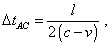(8)
 and for the ray reflected from B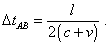(9)
 Thereupon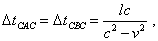(10)
 i.e. both rays will arrive to the observer in the rod middle simultaneously and during the time less than it would need if the rod were at rest with respect to the RF. And again, as the fact of rays return to the observer in the middle of rod is the event, the phase equality has to be registered by both stationary observers and moving observer, irrespectively of transformation of the space-time metric. It immediately follows from this analysis that 1) The postulate of light speed constancy in relativistic conception contradicts the conclusions that follow from the application of this postulate to the particular physical problems. 2) The clocks synchronisation technique based on sending the signals between the mutually stationary clocks can be applied exceptionally in case of stationary RF, in reference not to another RF but to the aether as the luminiferous medium that Einstein deleted in his conception. When this condition was violated, it appears that in absence of luminiferous aether all RFs have to have the anisotropy of light speed that arbitrarily depends on, with which RF we will compare. And if we have two or more RFs to compare, for each case we will yield incompatible values. 3) The yielded results evidence that the anisotropy of light speed is caused not by reduction of the rod either of the space-time metric but by the features of RF’s motion. This is like Ptolemy measured the epicycloids premising the Earth to be the centre, but this did not mean that planets really moved along these trajectories, though from the view of terrestrial observer these epicycloids gave quite accurate predictions of planets location in time. Before finishing this issue, we would like to mark, this result is natural from the view of classical physics. Having postulated the constancy of light speed in all inertial RFs, Einstein could not escape the physical reality and sequentially encountered the discrepancy of the introduced postulates, disproving them in turn, as we showed it in our paper “On black holes reality”. In fact, we showed it also in a trivial mental experiment with the moving rod. And should Einstein in his first work care of correspondence in his postulates and physical phenomena, we would need not so much time and efforts of many generations of physicists to get over the mistakes. The formulas (4) and (6) for the problem similar to that which we considered here, Einstein yielded in the very first paper of 1905 [3, p. 12] but did not conclude that the non-simultaneity contradicts the basic postulate of light speed constancy; he confined himself to the following remark. “Thus, we see, we should not attach absolute importance to the concept of simultaneity. Two events simultaneous for the observer of one coordinate system are not taken already as simultaneous when observed from the frame moving in relation to this first” [3, p. 13]. In this way he came to a contradiction with his own statement that the event is event for all RFs having observers at the given moment of time and given point of space. Here he started his ascendance from one mistake to another, from one discrepancy to another.

Contents: / 1 / 2 / Paper